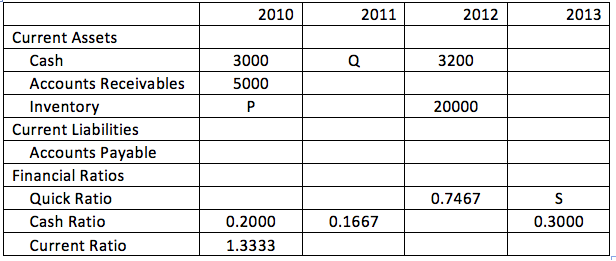## Data Interpretation Test 1

Instructions

The liquidity analysis of a company over the years 2010-13 is shown in the table. The table gives some of the values for Current Assets (made up of Cash, Accounts Receivables and Inventory) and Current Liabilities (Accounts Payable). The Financial Ratios given in the table help us understand the liquidity position of the company i.e. whether the company will be able to meet its short-term obligations with its short-term assets. The formulae for the Financial ratios are as follows :
Quick Ratio = (Cash+ Accounts Rec)/(Acc Payable),
Cash Ratio = Cash/ (Acc Payable),
Current Ratio = (Cash+ Accounts Rec+Inventory)/(Acc Payable).
The Accounts payable were constant over the years 2010-2013.Q 1

What is the difference P-Q=?

Q 2

What was the Quick Ratio in 2010?

Q 3

If Accounts recievable was constant over 2012-13, what is the value of S?

Q 4

If the difference between Quick Ratio and Current Ratio in 2012 is 1.3333 then how much is the Accounts Receivables in 2012? (Put 0 as answer if it cannot be determined)

Backspace
789
456
123
0.-
Clear All
Q 5

If Accounts Recievables were constant in 2010-2011 then what is the Current Ratio for 2011?